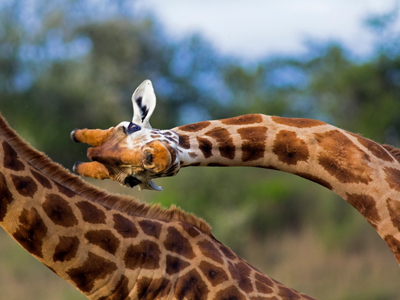A tree stands 5.47 metres tall. How tall would a giraffe need to be to see over the tree?

# Level 3-4 Numbers - Decimals - Ordering

Comparing and ordering numbers is useful when listing them by size. A good understanding of the value of a figure means you can compare one number with another and put several numbers in order. This KS3 Maths quiz will help you get the hang of ordering decimals.

Decimals are used for parts of numbers that are less than 1. When you put decimals in order, first compare the whole numbers, then the tenths, then the hundredths and then the thousandths. Unlike the numbers you are most used to, the value of numbers after decimal points goes down instead of up. That means that 0.099 is worth less than 0.1 - 1 tenth is more than 0 tenths, 9 hundredths and 9 thousandths.

Practise ordering decimals by playing this quiz. It may seem an easy task but take care! Read each question and look hard at the answers before you choose which one is right. Overconfidence can lead to careless mistakes!

1.
A tree stands 5.47 metres tall. How tall would a giraffe need to be to see over the tree?
5.04 metres
5.047 metres
5.74 metres
5.417 metres
Any number above 5.47 could be right so the only possible answer given is 5.74
2.
Which of these is the smallest number?
47.8
48.7
40.7
4.078
First compare the whole numbers!
3.
What comes next: 1.2, 1.25, 1.3, 1.35?
1.04
1.39
1.4
1.45
The sequence is increasing by 0.05 each time
4.
Which of the following numbers is the second largest?
3.4
3.14
3.41
3.1
The order from smallest to largest is 3.1, 3.14, 3.4 and 3.41
5.
Which of these is the largest number?
96.7
9.67
97.6
9.76
Look at the numbers before the decimal point first
6.
My television cable is 4.3m, my mobile phone charger is 3.2m, my PlayStation lead is 4.13m and my hi-fi lead is 2.78m. Which is the longest?
Television cable
Mobile phone charger
4.3 is the largest number on the list
7.
A rollercoaster at the amusement park has a sign that says you must be over 1.1 metres to ride it. Which of these heights is unable to ride?
1.12 metres
1.07 metres
1.2 metres
1.31 metres
1.07 is less than 1.10
8.
Which of these is the smallest number?
2.5
2.15
2.17
2.8
It helps to give the numbers the same number of decimal places by adding zeros, e.g. 2.50 and 2.80
9.
Colin gets £2.40 pocket money, Michelle gets £2.15, Geoff gets £2.75 and Sarah gets £2.55. Who gets the most?
Colin
Michelle
Geoff
Sarah
One penny is a hundredth of a pound so would be written as £0.01
10.
Which of these is ordered from largest to smallest?
3.45, 3.5, 3.15, 3.55
3.5, 3.55, 3.45, 3.15
3.15, 3.45, 3.5, 3.55
3.55, 3.5, 3.45, 3.15
Look for the highest number in the tenths column
You can find more about this topic by visiting BBC Bitesize - Place value and ordering decimals

Author:  Frank Evans